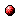# Urban traffic control using a fuzzy multi-agent system

Reference:
A. Jamshidnejad, B. De Schutter, and M.J. Mahjoob, "Urban traffic control using a fuzzy multi-agent system," Proceedings of the 2015 European Control Conference, Linz, Austria, pp. 3046-3051, July 2015.

Abstract:
This paper presents a fuzzy multi-agent system for control of the traffic signals of an urban network, where the aim is to reduce the total average delay time of the vehicles. The large-scale traffic system is first divided into sub-areas and an agent, using a fuzzy controller, is considered for each sub-area. In order to develop the fuzzy rule bases for the agents, an extensive set of data is collected and the corresponding origin-destination (OD) matrices are calculated. The OD matrices are then clustered using a new clustering algorithm proposed in this paper. Finally, the resulting cluster centers, which are matrices of the same dimension as the OD matrices, are mapped to a 2-dimensional space and the corresponding triangular fuzzy sets are extracted. An optimal cycle plan is found for each fuzzy set and this way the fuzzy rule bases are constructed (the triangular fuzzy sets form the IF-parts and the optimal cycle plans form the THEN-parts). In a case study the proposed multi-agent control system is applied to a microscopic urban traffic network and the results are compared with a controller that applies optimal signal plans calculated by PASSER V. The results show that the proposed fuzzy multi-agent system outperforms the non-fuzzy control system, where the average delay time of the traveling vehicles is decreased by 19% using the multi-agent control system.Corresponding technical report: pdf file (202 KB)
Note: More information on the pdf file format mentioned above can be found here.

Bibtex entry:

@inproceedings{JamDeS:15-007,
author={A. Jamshidnejad and B. {D}e Schutter and M.J. Mahjoob},
title={Urban traffic control using a fuzzy multi-agent system},
booktitle={Proceedings of the 2015 European Control Conference},